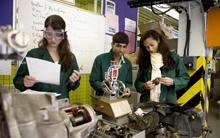# STEM: Lou-Vee Air CarLesson Plans

PostLou-Vee-Air Car is a sample STEM lesson created by a CTE and math teacher team from Utah. The lesson’s objective is to teach students to identify the correlation between the mass of a car and the acceleration the car is experiencing and then recognize the action/reaction forces at work. The CTE concepts taught in this lesson are about energy, mass, and weight, including Newton’s second (force = mass * acceleration, or F = ma) and third (action/reaction) laws of motion. Math concepts include formulas, identification of variables, equations, and graphing variables.

Math-enhanced CTE lessons are written only after CTE and math teacher teams work together to develop a curriculum map that identifies the CTE concepts and intersecting math concepts (including the Common Core math standards) that are naturally embedded within the CTE curriculum. Once the map is complete, the CTE and math teacher teams, working in communities of practice, select from within it one or more CTE concepts and related math concepts to develop into CTE lesson plans. Math-in-CTE’s seven-element pedagogic framework (PDF) guides this lesson development process. When the lessons are complete, the CTE teachers create scope and sequence plans for integrating the lessons into their courses.